# SSC EXAMS | Reasoning Ability Practice Questions (Day-27)

Dear Aspirants, Here we have given the Important SSC Exam 2019 Practice Test Papers. Candidates those who are preparing for SSC 2019 can practice these questions to get more confidence to Crack SSC 2019 Examination.

[WpProQuiz 5335]

1) Complete the following series on a certain logical way

M Y 0 3, I L 0 2, N P 0 2, K T ?

a) 09

b) 08

c) 07

d) 06

2) B @ E = 9; D * F = 35; A G = – 6; M \$ F = 2, then find the value of ‘H \$ D * I @ A B ?

a) 17

b) 16

c) 30

d) 40

3) From the given answer figures, select the one in which the question figure is hidden/ embedded.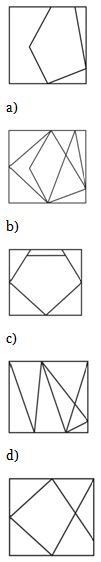4) Which of the following cube in the answer figure cannot be made based on the unfolded cube in the question figure?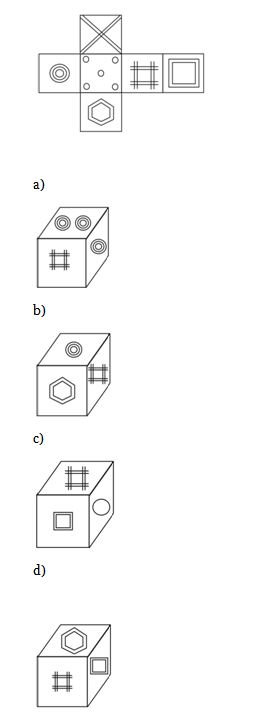5) Complete the series of the given pattern?

(STAR ; 85); (MOON ; 55) ; (SUN; ?)

a) 54

b) 45

c) 20

d) 90

6) Select the similar relations between given terms in the options

K E Y : E : : L O C K : ?

a) F

b) E

c) G

d) D

7) Consider the following statement and answer the question.

W, X, Y & Z are all different individuals W is the daughter of x. Y is the father of x. Z is the brother of W. What is the relation between ‘Z’ and ‘Y’?

a) Relation can’t be established

b) Grand Father

c) Grand Mother

d) None of these

8) What is the next letter in the series?

M, P, T, Y ……?

a) E

b) D

c) F

d) G

Directions (9 – 10): Find odd one out from all the given options.

9)

a) Graphite

b) Aluminum

c) Diamond

d) Silver

10)

a) Oxygen

b) Ozone

c) Nitrogen

d) Potassium

MY = M (13) Y (25) = 25 – 13 = 12 = 1 + 2 = 03

J L = J (10) L (12) = 12 – 10 = 02

NP = N (14) P (16) = 16 – 14 = 02

KT = K (11) T (20) = 20 – 11 = 09

B @ E = (2 + 1) + (5 + 1) = 3 + 6 = 9

D * F = (4 + 1) × (6 + 1) = 5   7 = 35

A र G = (1 + 1) – (7 – 110 = 2 – 8 = – 6

M \$ F = (13 – 11)  (6 + 1)= 14 ÷  7 = 2

So, H \$ D * E @ A र B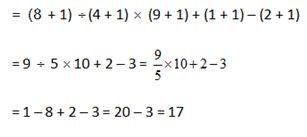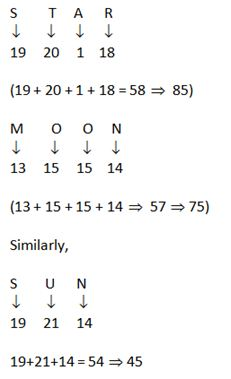KEY = 11 + 5 + 25 = 41 = 4 + 15 (E)

Similarly, LOCK = 12 + 15 + 3 + 11 = 41 = 4 + 1 = 5 (E)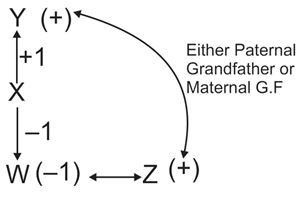Gapping of letters is continuously in creating as + 2, + 3, + 4, + 5 and so on.

Hence, D is required letter after y.

Directions (9-10):

‘Diamond’ is odd here because rest of diamond all is good conductor of electricity.

Potassium is metal and all the three are non-metal also gases.